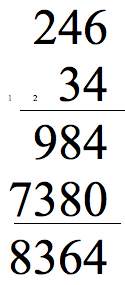#### You may also like### Exploring Wild & Wonderful Number Patterns

EWWNP means Exploring Wild and Wonderful Number Patterns Created by Yourself! Investigate what happens if we create number patterns using some simple rules.### Sending Cards

This challenge asks you to investigate the total number of cards that would be sent if four children send one to all three others. How many would be sent if there were five children? Six?### Dice and Spinner Numbers

If you had any number of ordinary dice, what are the possible ways of making their totals 6? What would the product of the dice be each time?

##### Age 7 to 14 Challenge Level:

These submissions show some interesting thoughts about these different methods, firstly Pablo St.Gregory's school wrote:

It will help if you watch someone do a math calculation before you answer it so you understand what it's telling you. They sort of all have the same calculation but it's just laid out differently.

 65 65 + 65 X 4 65 ----- 65 260 ----- 260

I would rather these ways than any others because it's quicker.

Keeley from St Blaise sent in the following:

I think the lines are the better solution because it is very easy to understand  because you put how many lines that you need. Say there was the number two, you put two lines.

Robbie from Middleton Tyas C of E Primary wrote:

I find that they are all useful. The one that I understand the most is the column multiplication because that is how I do it in my head. I find it helpful being able to do the addition at the same time as doing it which you can't do in any of the other methods. I find the multiplying with lines the hardest because I don't know where to put the lines.

Here is what Cleon and Alex from St.Blaise School sent in:

With the line method it works because it is split into separate sections.  The amount of dots in the section is part of your answer.

Valentins from Westfield Middle School said:

I think the column method is the best method because as I have tried doing every other method and iI think this is the best one as I worked out the answer the fastest and got it right.Chloe from Landau Fort Academy performed the same calculations on a different sum to make sure she had understood the methods, and added her thoughts on what she had done:

Grid method: easy, but takes ages to draw.

Column method: easy, but might get mixed up.

Multiplying with lines: doesn't take long once drawn, but it's quite hard!

Gelosia method: makes sense, but you might mix it up, and it takes a while to draw.

Holly, from Hymers College, gave some great explanations of the different methods:

The grid multiplication method works by splitting up the numbers (e.g. 23 into 20 and 3) to times them together more easily (as it is much easier trying to do 20x20 than 23x21). After you times every digit in the first number by every digit in the second number, you simply add them all up.

Column multiplication works in a similar way to the grid multiplication method, as the column method splits the numbers up in a similar fashion.

With the sum 23x21:
you do 1x3, and put the answer in the units column,
then 1x2, and put the answer in the tens column (you are basically doing 1x20 and carrying over from the units to the tens).
For the next line, as you are really doing 20x3, you put a 0 in the units column, and multiply 2x3; the 6 in the tens column represents 60.

The line method works so that after you have drawn all your lines and added them up, they are split into units, tens, hundreds, and so on. This is done by multiplying the units by units (the only way to get units), the tens by units and units by tens (the only ways to get tens), the units by hundreds and the tens by tens (the only ways to get hundreds), and so forth for any
other columns.

The Gelosia method still works with the units x units, tens x units, etc. system explained for the line method of multiplication. The squares are halved diagonally in case you need to 'carry' a digit over to the next column; it will simply be added in with the next column automatically, instead of you having to carry it over yourself.

Alexander from Wilson's School also gave similar explanations, and added his thoughts:

There are a few disadvantages to each of the methods:
Firstly, in Grid Multiplication, you have to do many multiplication sums, and then add them all together.
In column multiplication, the problem is that if you forget to add the zero in the second row, your product will be incorrect.
Line multiplication takes a long time to draw and can get very confusing.
Gelosia multiplication's disadvantage is that it takes a long time to draw, and if you've got a big sum, you have to carry a lot of numbers.

However, all of the methods have advantages:
Grid Multiplication method is very simple, therefore very difficult to get wrong.
Column multiplication is good because it doesn't take up that much space and is very quick.
In Multiplying with lines you don't have to multiply, you just count.
Gelosia multiplication is good because it carries most of the numbers that have to be carried for you.

Well thank you for your thoughts about these different methods, it's good to think about the different ways that different people work things out.